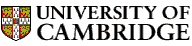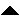Computer LaboratoryComputer Science Syllabus - Numerical Analysis IINumerical Analysis IINext: Easter Term 2006: Part Up: Lent Term 2006: Part Previous: Introduction to Functional Programming   Contents

## Numerical Analysis II

Lecturer: Dr M.R. O'Donohoe

No. of lectures: 12

Prerequisite course: Numerical Analysis I

Aims

This course will build on the foundation of Numerical Analysis I and provide more mathematical analysis to cover a much wider range of problems and techniques. It will blend theory and practice in order to provide a usefully broad body of knowledge.

Lectures

• Elementary approximation theory. Polynomial approximation; Taylor series; interpolation; best approximations; Chebyshev polynomials; Chebyshev series; economisation; least-squares approximation; the Gram-Schmidt process; range reduction; square roots; splines. [3.7 lectures]

• Quadrature. Riemann sum rules; calculation of weights; error analysis; Gaussian quadrature; composite rules; singular integrals; multi-dimensional integration; standard regions; product rules; Monte Carlo methods. [2.3 lectures]

• Non-linear equations and optimisation. Non-linear equations in one variable; simple iterative methods; fixed-point iteration theory; zeros of polynomials; unconstrained optimisation; Newton methods; steepest descent methods. [2 lectures]

• Numerical linear algebra. Calculation of eigenvalues and eigenvectors; effective rank of a matrix; generalised inverse matrices; singular value decomposition; matrix norms; condition of linear equations. [2 lectures]

• Differential equations. Initial value problems; Euler's method; Runge-Kutta methods; multistep methods; predictor-corrector methods; stability theory; stiff systems. [2 lectures]

Objectives

At the end of the course students should

• have an understanding of basic approximation theory and its extension to quadrature, fixed-point iteration theory, and elementary stability theory for numerical solution of initial value ordinary differential equations

• be able to apply several advanced numerical methods, such as singular value decomposition

• be able to make an informed choice, in several problem areas, between diverse methods commonly available in numerical software libraries© 2005 University of Cambridge Computer Laboratory Please send any comments to pagemaster@cl.cam.ac.uk Page last updated on 11-Sep-2005 at 15:57 by Christine Northeast